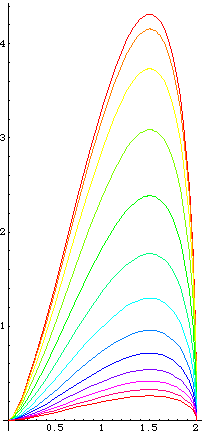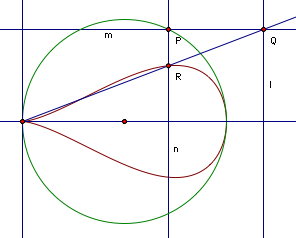# PiriformA family of piriform curves. (the symetric bottom halves are not shown).

Mathematica Notebook for This Page

## Description

Piriform is a family of curves with one parameter. They are tear-drop shaped.Piriform Construction

Let there be circle centered on {1,0} with radius 1. Let l be a line parallel to y-axes h distant to the right. Let P be a point on the circle. let m be a line passing P and parallel to x-axis. Let n be a line passing P and parallel to y-axis. Let Q be the intersection of m and l. Let R be the intersection of n and line from Origin to Q. The trace of R as P moves around the circle is the Piriform, with parameter h.

## Formula

Parametric: {1,Sin[t]/h} * (1+Cos[t]). Period is 2 π.

2006-05

If you have a question, put \$5 at patreon and message me.